# MATHEMATICS END OF TERM 1 YEAR 11 PROGRESSIVE TEST DECEMBER 2021

Created
Best for live in-class or video conferencing lessonsStart teacher-led lesson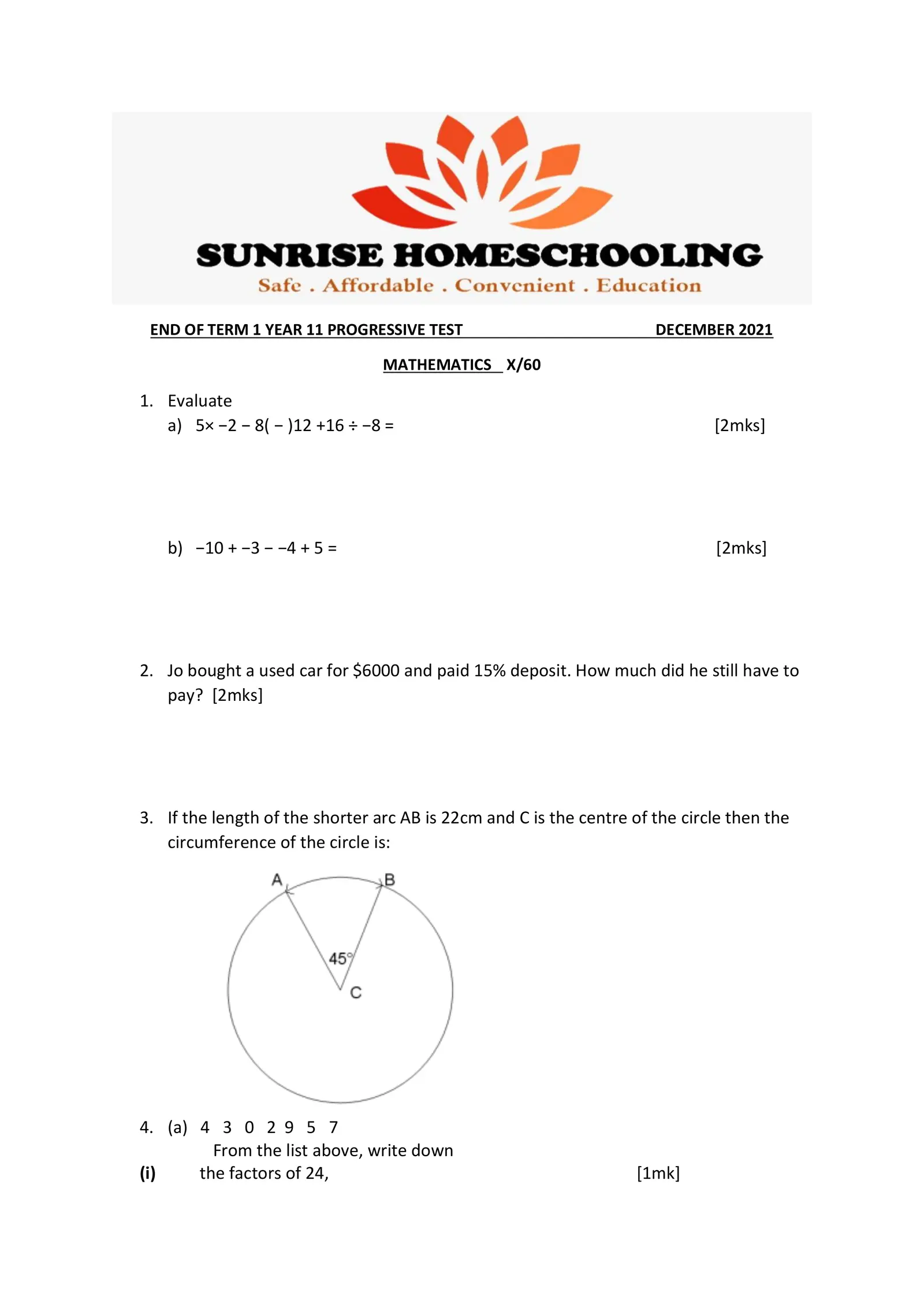END OF TERM 1 YEAR 11 PROGRESSIVE TEST DECEMBER 2021 MATHEMATICS X/60 1. Evaluate a) 5× −2 − 8( − )12 +16 ÷ −8 = [2mks] b) −10 + −3 − −4 + 5 = [2mks] 2. Jo bought a used car for \$6000 and paid 15% deposit. How much did he still have to pay? [2mks] 3. If the length of the shorter arc AB is 22cm and C is the centre of the circle then the circumference of the circle is: 4. (a) 4 3 0 2 9 5 7 From the list above, write down (i) the factors of 24, [1mk]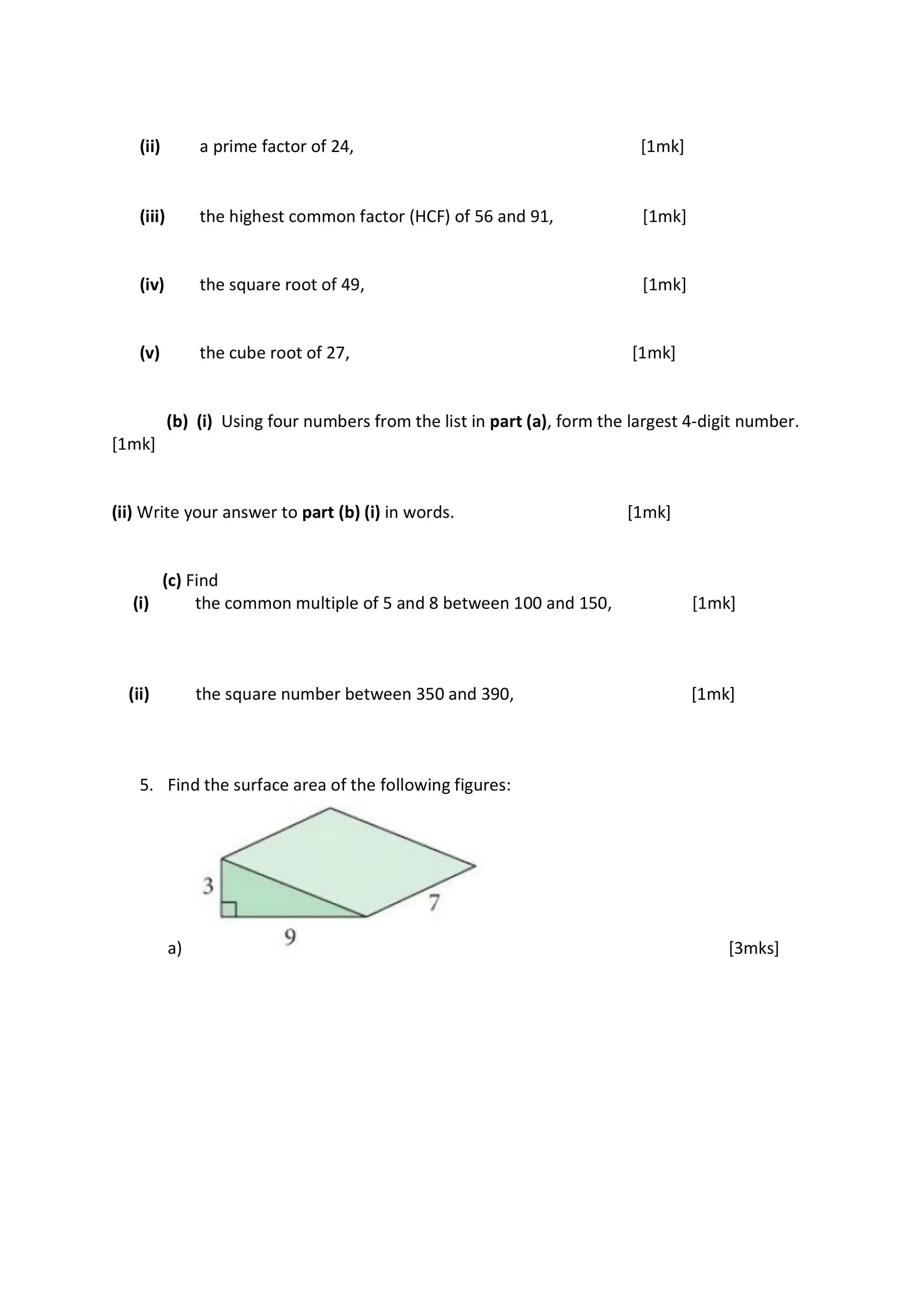(ii) a prime factor of 24, [1mk] (iii) the highest common factor (HCF) of 56 and 91, [1mk] (iv) the square root of 49, [1mk] (v) the cube root of 27, [1mk] (b) (i) Using four numbers from the list in part (a), form the largest 4-digit number. [1mk] (ii) Write your answer to part (b) (i) in words. [1mk] (c) Find (i) the common multiple of 5 and 8 between 100 and 150, [1mk] (ii) the square number between 350 and 390, [1mk] 5. Find the surface area of the following figures: a) [3mks]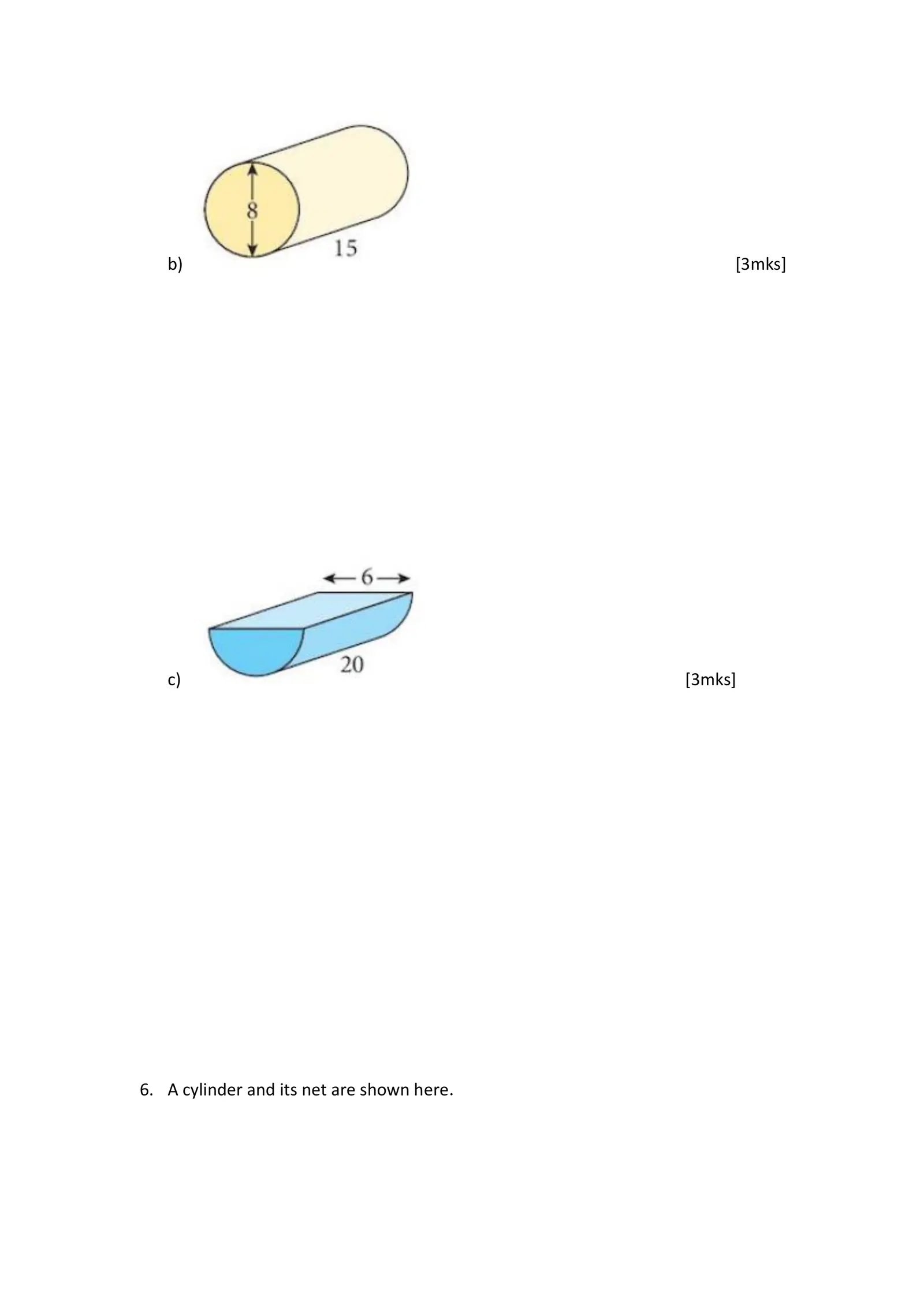b) [3mks] c) [3mks] 6. A cylinder and its net are shown here.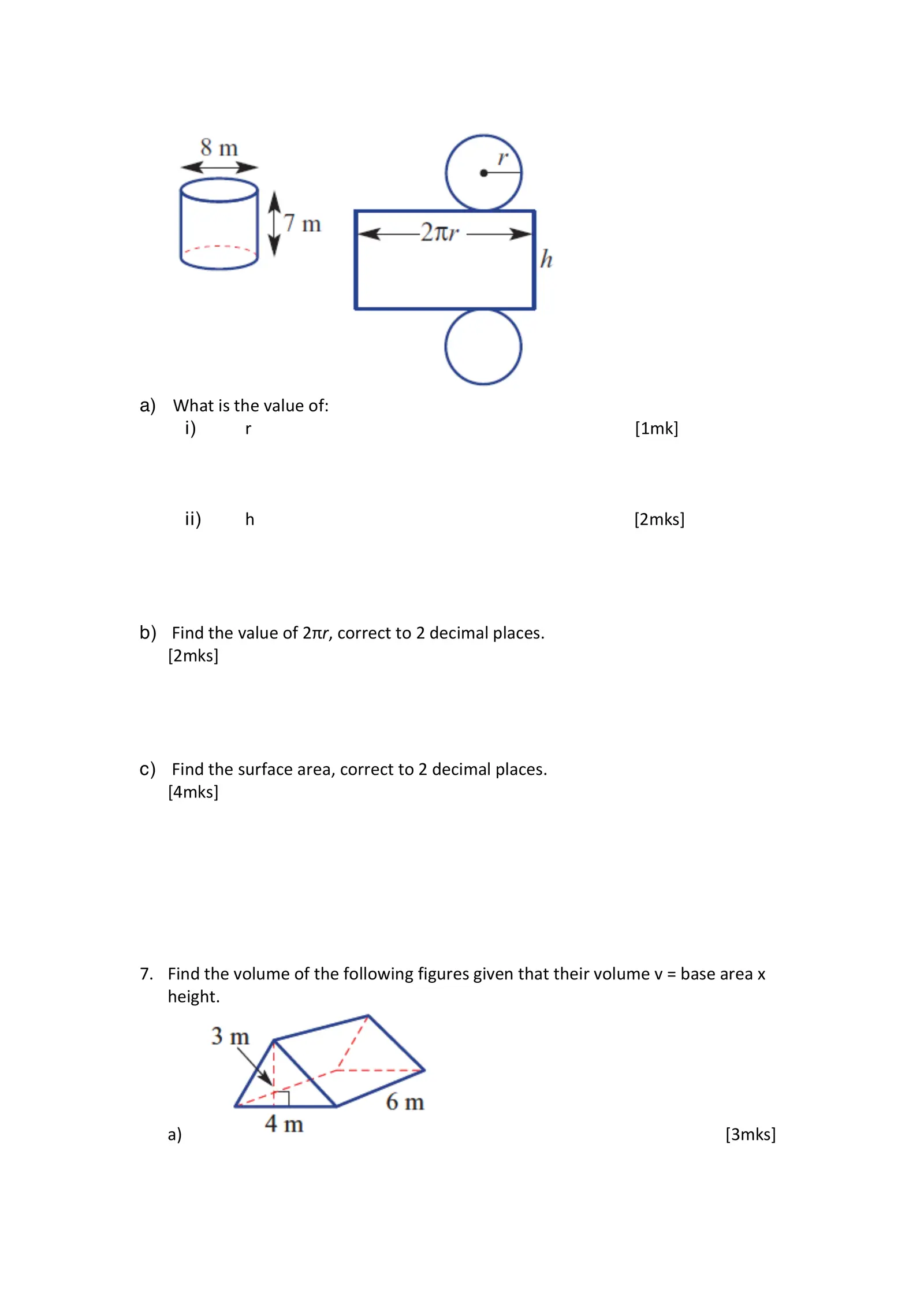a) What is the value of: i) r [1mk] ii) h [2mks] b) Find the value of 2πr, correct to 2 decimal places. [2mks] c) Find the surface area, correct to 2 decimal places. [4mks] 7. Find the volume of the following figures given that their volume v = base area x height. a) [3mks]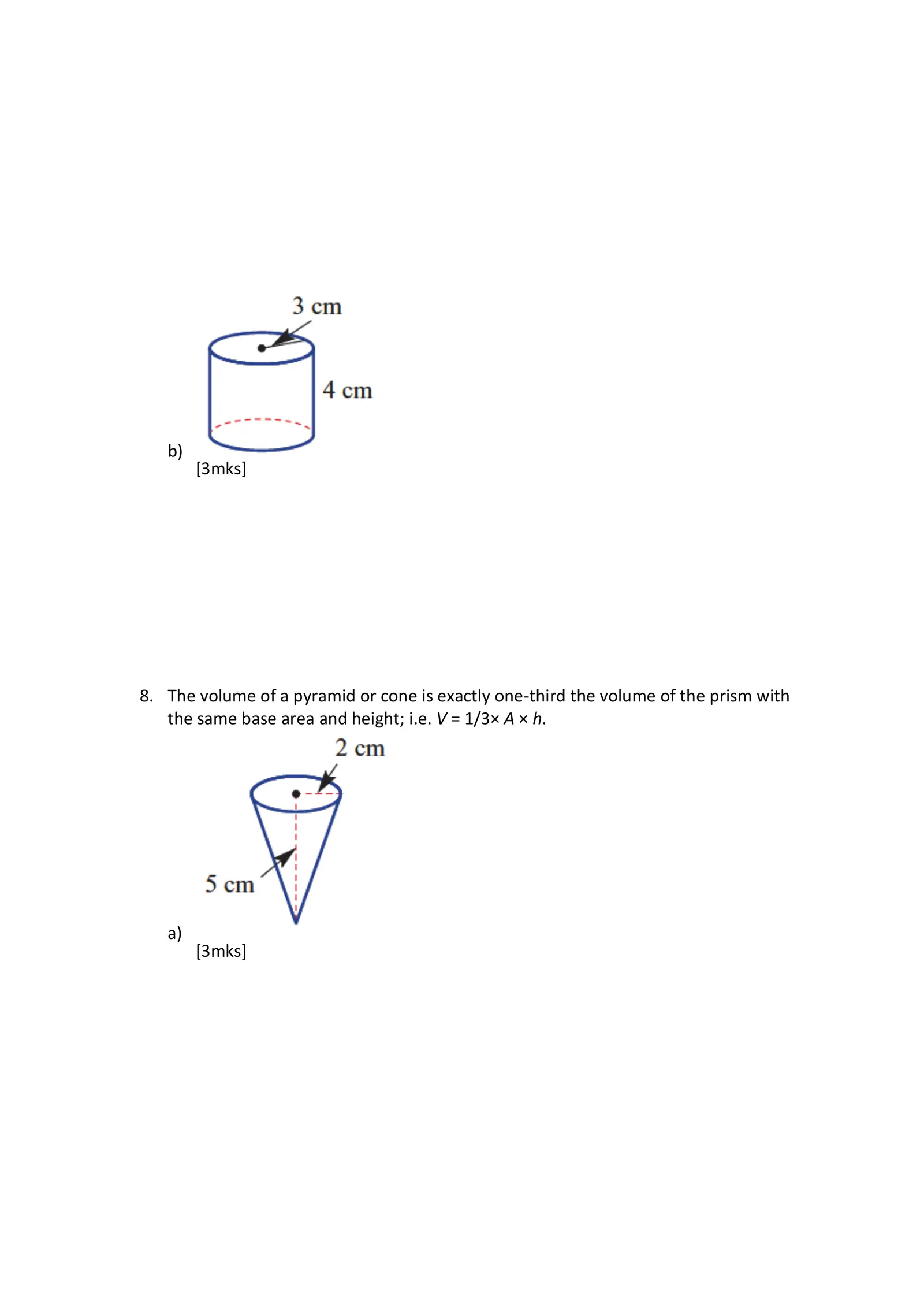b) [3mks] 8. The volume of a pyramid or cone is exactly one-third the volume of the prism with the same base area and height; i.e. V = 1/3× A × h. a) [3mks]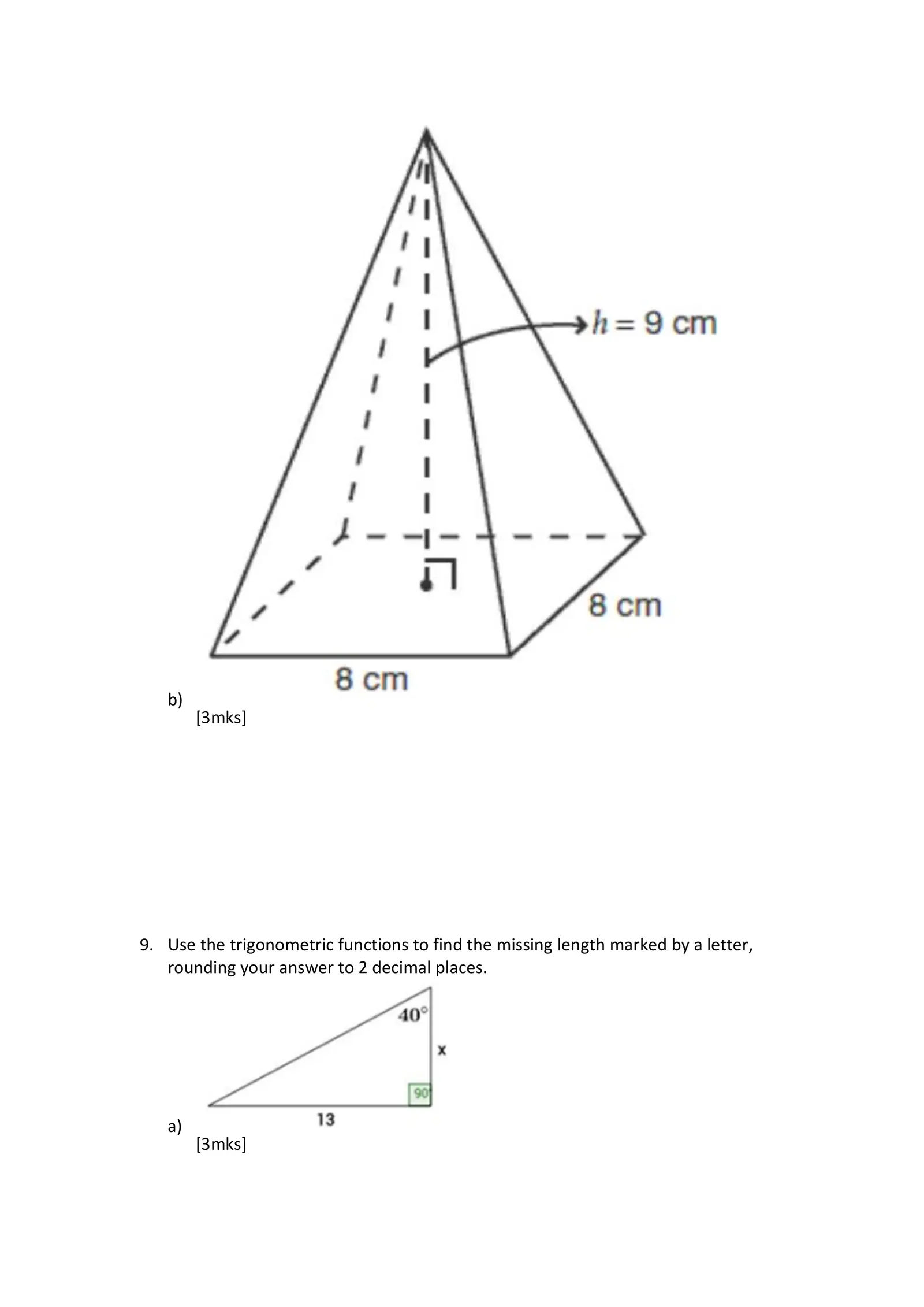b) [3mks] 9. Use the trigonometric functions to find the missing length marked by a letter, rounding your answer to 2 decimal places. a) [3mks]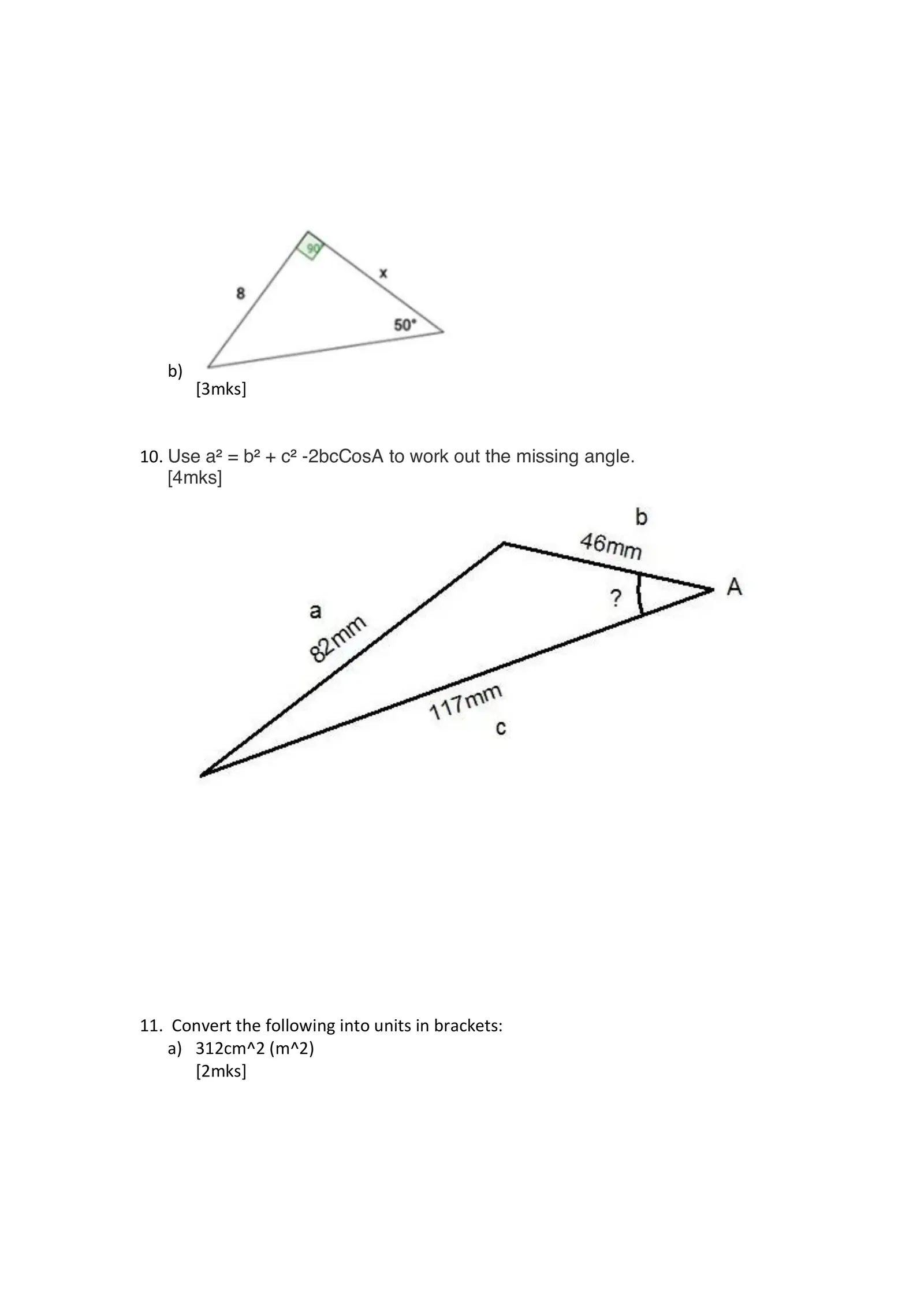b) [3mks] 10. Use a² = b² + c² -2bcCosA to work out the missing angle. [4mks] 11. Convert the following into units in brackets: a) 312cm^2 (m^2) [2mks]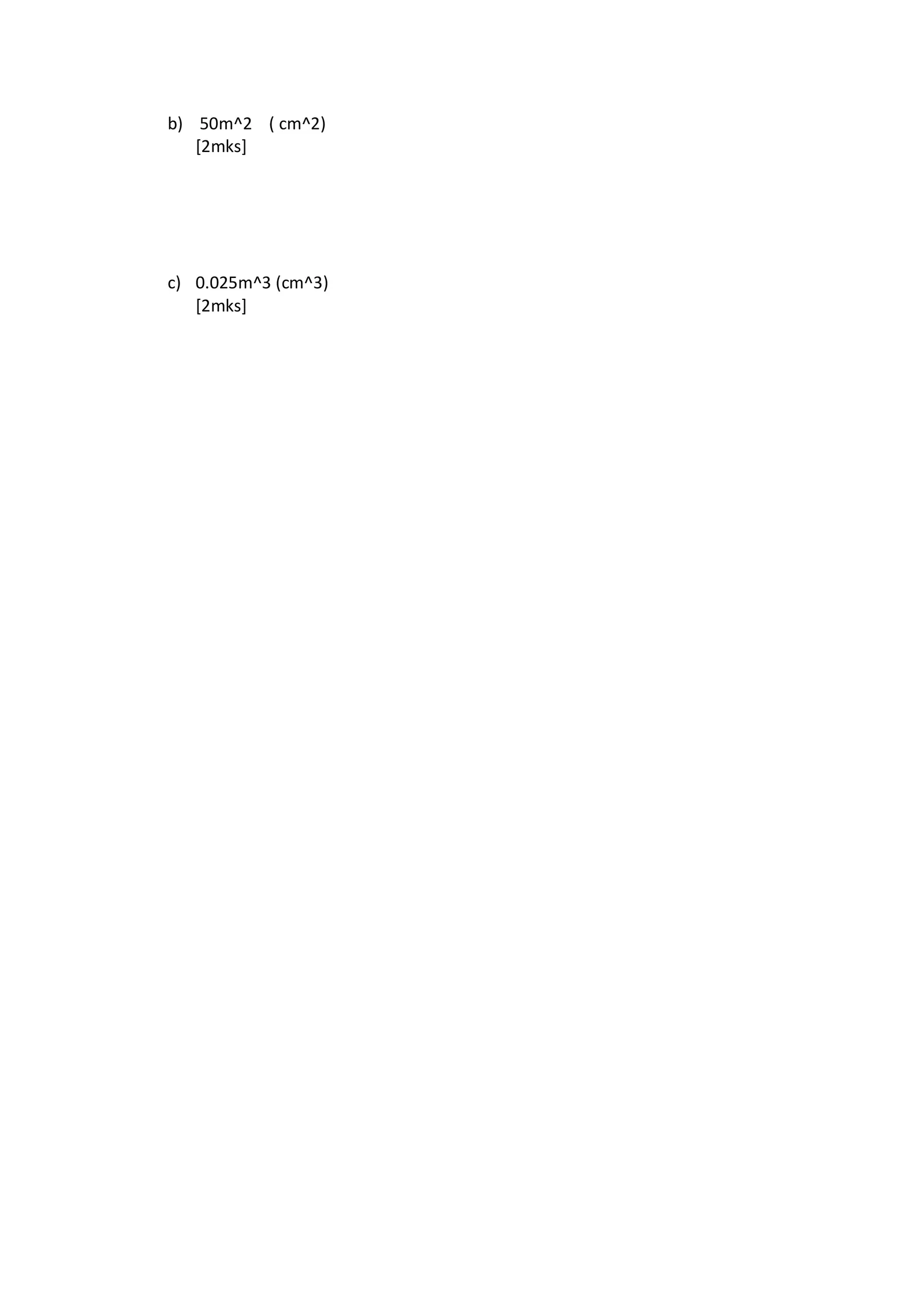b) 50m^2 ( cm^2) [2mks] c) 0.025m^3 (cm^3) [2mks]